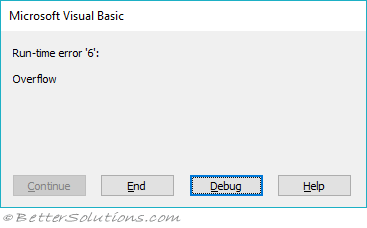# Integer

The integer data type can contain any number between -32,768 and 32,767.
This data type uses 2 bytes.
One byte is used to represent the sign (either positive or negative).
The default value is 0.

`Dim myInteger As Integer `

### Integer is converted to Long

On 32-bit systems the Integer data type is implicitly converted to a Long data type.
However this conversion does not mean that a larger range can be accepted.
If you declare an Integer data type it can still only contain a number between -32,768 and 32,767.
For this reason there is no point declaring any variables with an Integer data type.
There used to be a performance advantage to using Integer over a Long but that is no longer the case.
In fact using a Long data type might actually be faster than using an Integer data type because there is no implicit conversion.
It is worth noting though that some old API functions may still expect an Integer data type.
If you pass a Long (4 bytes) when it is expecting an Integer (2 bytes) it will not work due to the different byte sizes.

The following code will generate an error.

`Dim myInteger As Integer myInteger = 40000 Call MsgBox(TypeName(myNumber)) `### Conversion Function

The CINT function returns an expression converted to an integer data type.